# Static and Global Variables in Recursion

## Static and Global Variables in Recursion with Examples

In this article, I am going to discuss Static and Global Variables in Recursion with Examples. Please read our previous article where we discussed the time complexity of Recursive Functions.

##### Static and Global Variables in Recursion

Let us see how static variable works with recursive function with an example. We have seen how to trace recursion in our previous article. We have also seen how a recursive function utilizes a stack. Then the question is what will happen when the variables are static i.e. how to treat the static variables inside a recursive function.

The following is an example of a recursive function, and there are no static variables right now. Without static variables, I will show you how to trace it and when static is introduced then I will show you how to trace it and what should be the difference. But before that, first, let us see what the following function is doing.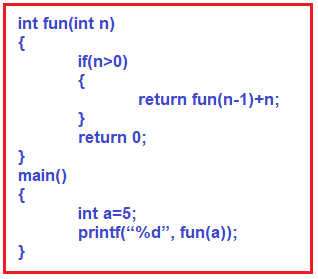As you can see in the above image, there is a function called fun which takes parameter n of type integer. Then if the n value is greater than 0, it will call itself with a decreased value of n (i.e. n – 1) and also add n. So, when this plus n (i.e. +n) will be done (calling time or returning time)? It is done at returning time. If the value of n is 0, then it returns 0. From the main function, we called the fun function passing ‘a’ i.e. 5. Let us trace the above recursive function. The following image shows the tracing of the fun call.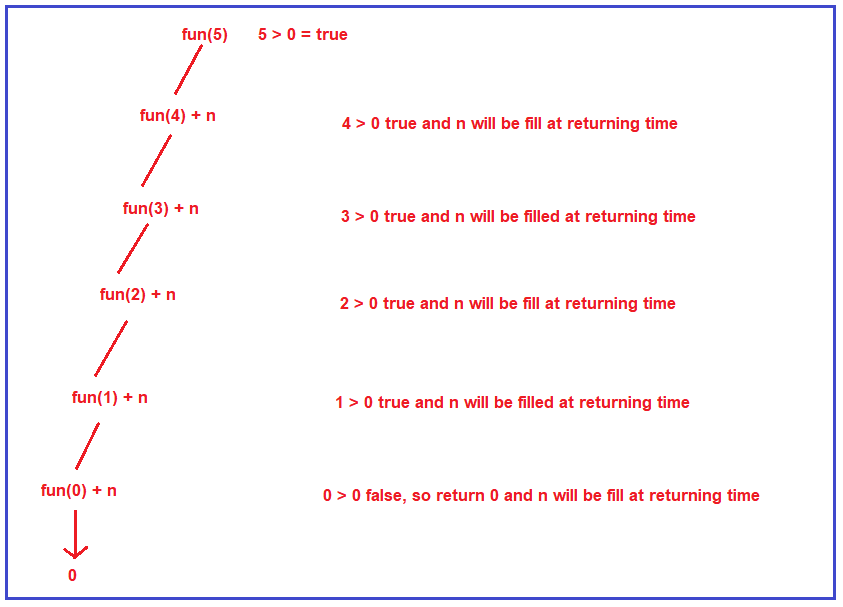First, the function fun is called for the value 5 & 5 is greater than 0. Yes, so it will call itself with the reduced value of n i.e. 4, and n i.e. 5 will be added at returning time. Then it will check whether 4 is greater than 0, yes, so it will call itself again with a reduced value of n i.e. 3 and the current n value i.e. 4 will be added at returning time. In this way, it will itself until the “n” value becomes 0. When the n value becomes 0, the condition becomes false and it will not call itself rather it simply returns 0. From this onward the returning will happen and to the result of each function call, the n value will be added. For better understanding, please have a look at the below image.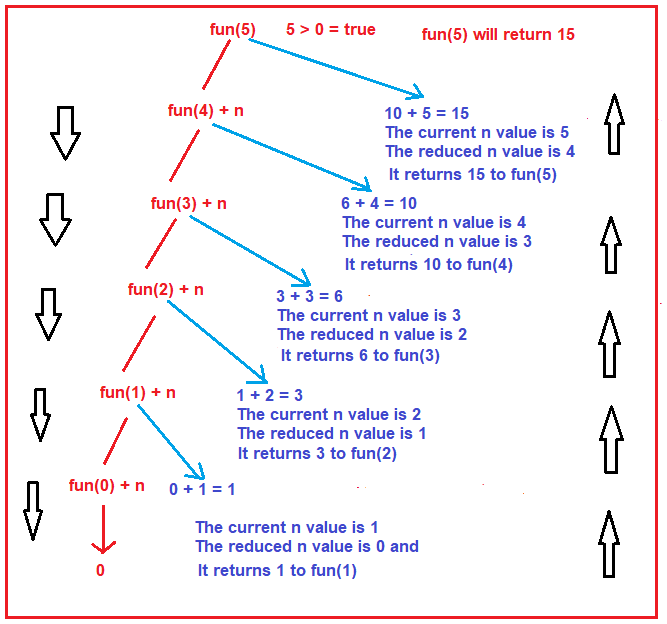Let us understand how the returning will happen step by step

Fun(0) + n: In this case, the current n value is 1 and the reduced n value is 0 and fun(0) will return 0 and the current n value i.e. 1 will be added with the result of fun(0). So, this will returns 1 to the previous function call i.e. fun(1) i.e. the result of the fun(1) function will be 1.

Fun(1) + n: In this case, the current n value is 2 and the reduced n value is 1, and fun(1) returns 1 (the output of previous function call) and the current n value i.e. 2 will be added with the result of fun(1). So, this will returns 3 to the previous function call i.e. fun(2) i.e. the result of the fun(2) function will be 3.

Fun(2) + n: In this case, the current n value is 3 and the reduced n value is 2, and fun(2) returns 3 (the output of previous function call) and the current n value i.e. 3 will be added with the result of fun(2). So, this will returns 6 to the previous function call i.e. fun(3) i.e. the result of the fun(3) function will be 6.

Fun(3) + n: In this case, the current n value is 4 and the reduced n value is 3, and fun(3) returns 6 (the output of previous function call) and the current n value i.e. 4 will be added with the result of fun(3). So, this will returns 10 to the previous function call i.e. fun(4) i.e. the result of the fun(4) function will be 10.

Fun(4) + n: In this case, the current n value is 5 and the reduced n value is 4, and fun(4) returns 10 (the output of previous function call) and the current n value i.e. 5 will be added with the result of fun(4). So, this will returns 15 to the previous function call i.e. fun(5) i.e. the result of the fun(5) function will be 15.

So, at the end fun(5) will return 15. This is the tracing of the above function when it called with a value of 5. Now, let us see how the activation record is created. The activation record for the fun function will be created. For each value of n i.e. (5, 4, 3, 2, 1, 0) one activation record is created in the stack as shown in the below image. This is how the stack is created every time for each call.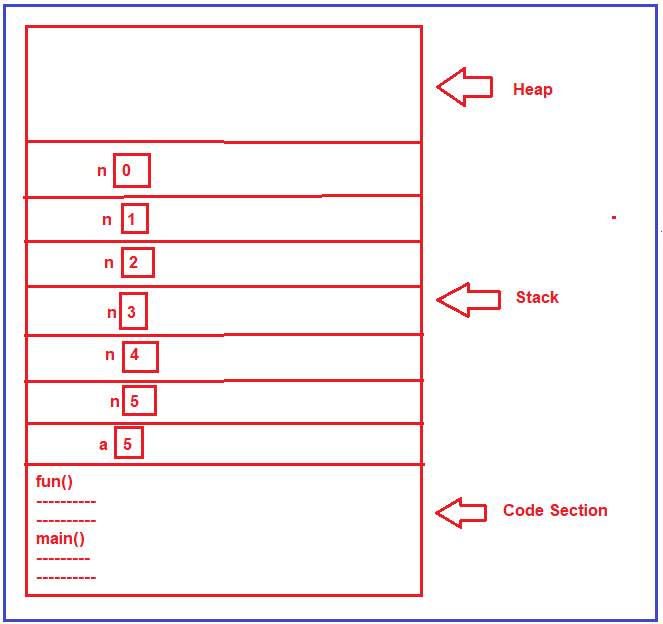##### Example: Without using Static and Global Variables

The following is the complete example code of recursion without static and global variables in C.

```#include <stdio.h>
int fun (int n)
{
if (n > 0)
{
return fun (n-1) + n;
}
return 0;
}

int main ()
{
int a = 5;
printf ("%d", fun(a));
return 0;
}
```

Output: 15

##### Static Variables in Recursion with Examples:

Now, we are going to make changes in the fun function and then we will see how to handle static variables. Here, we are creating a static integer variable (i.e. x) whose value is initialized with 0. Before calling the function itself, we are incrementing the static variable value by 1 and while returning we are adding x as shown in the below image.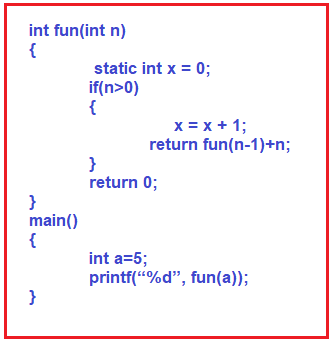First of all, we should know where static variables are created, they are created inside the code section or there is a subsection inside the code section called a section for global variables and static variables. But I am showing directly inside the code section and the value of that variable is 0 as shown in the below image.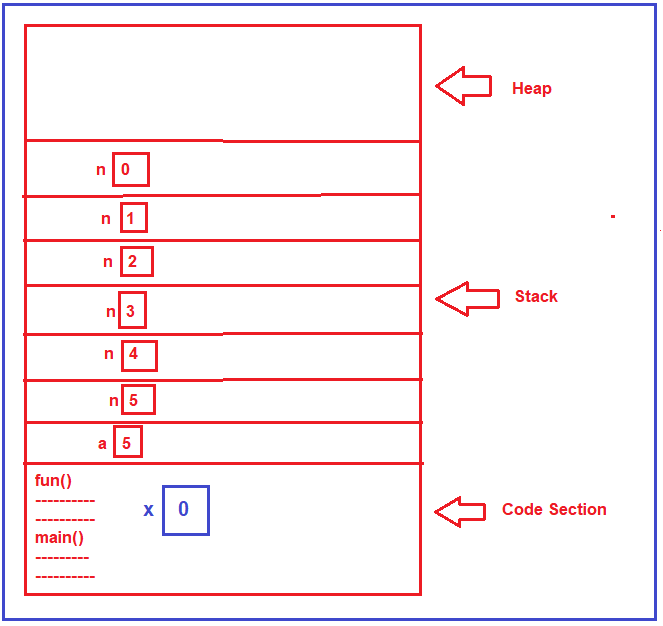Will be the static variable created every time whenever the function is called? No, it will not be created every time when the function is called. It is created only one time that is at the loading time of a program. This static variable x will not be having multiple copies just like n, it will only have a single copy. All the function calls will use the same copy of x, but for n every time a new copy is created.

Now, what will be the result of this function? For that, we will trace it again. Let us look at the tracing and see how static variables are handled in a recursive function.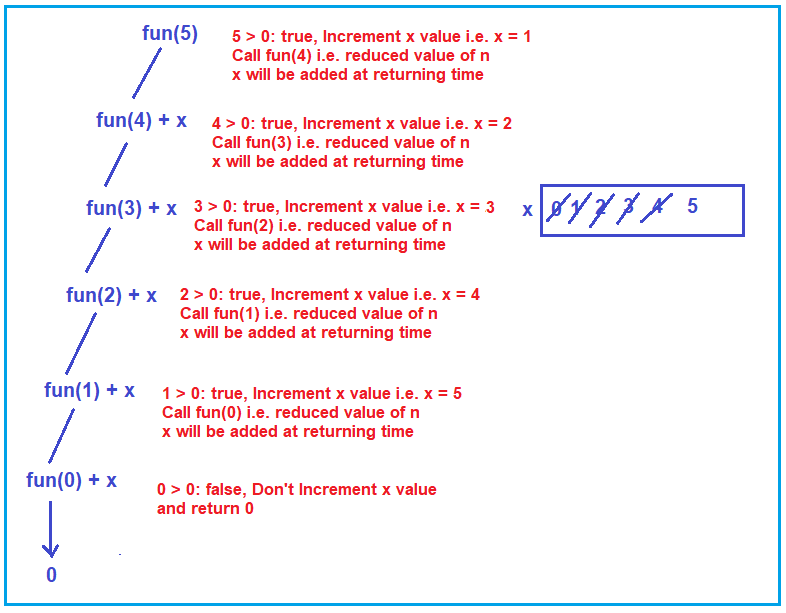First, the function fun is called for the value 5 & 5 is greater than 0. Yes, so it will increment the x value i.e. x becomes 1, and then it calls itself with the reduced value of n i.e. 4, and x will be added at returning time. Then for fun(4) function call, again it will check whether 4 is greater than 0, yes, so again it will increment the x value i.e. x becomes 2, and call itself again with a reduced value of n i.e. 3 and the x will be added at returning time. In this way, it will increment the x value and call itself until the “n” value becomes 0. When the “n” value becomes 0, at that time x value becomes 5, and that what you can see in the above image.

When n value becomes 0, the condition becomes false and it will not call itself rather it simply returns 0. From this onward the returning will happen and to the result of each function call, the x value will be added. The x value is 5 and that will be added in each step. For better understanding, please have a look at the below image.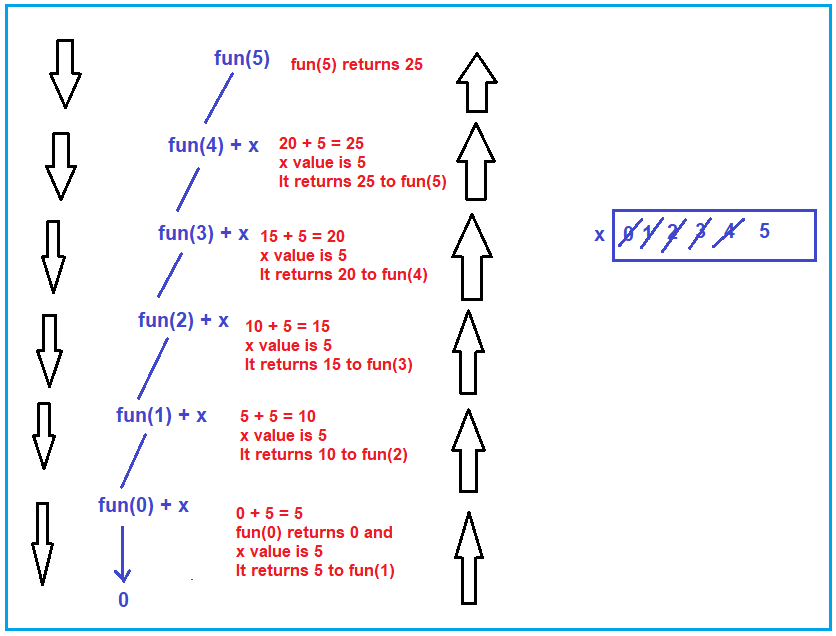Let us understand how the returning will happen step by step

Fun(0) + x: In this case, the current n value is 1 and the reduced n value is 0, and fun(0) will return 0 and the x value is 5 and that will be added with the result of fun(0). So, this will returns 5 to the previous function call i.e. fun(1) i.e. the result of the fun(1) function will be 5.

Fun(1) + x: In this case, the current n value is 2 and the reduced n value is 1, and fun(1) returns 5 (the output of previous function call) and x value is 5 and that will be added with the result of fun(1). So, this will returns 10 to the previous function call i.e. fun(2) i.e. the result of the fun(2) function will be 10.

Fun(2) + x: In this case, the current n value is 3 and the reduced n value is 2, and fun(2) returns 10 (the output of previous function call) and the x value is 5 and that will be added with the result of fun(2). So, this will returns 15 to the previous function call i.e. fun(3) i.e. the result of the fun(3) function will be 15.

Fun(3) + x: In this case, the current n value is 4 and the reduced n value is 3, and fun(3) returns 15 (the output of previous function call) and the x value is 5 and that will be added with the result of fun(3). So, this will returns 20 to the previous function call i.e. fun(4) i.e. the result of the fun(4) function will be 20.

Fun(4) + x: In this case, the current n value is 5 and the reduced n value is 4, and fun(4) returns 20 (the output of previous function call) and the x value is 5 and that will be added with the result of fun(4). So, this will returns 25 to the previous function call i.e. fun(5) i.e. the result of the fun(5) function will be 25.

So, at the end fun(5) will return 25. This is the tracing of the above function when it called with a value of 5 and using a static variable.

##### Example: Recursion using Static Variables in C
```#include <stdio.h>
int fun (int n)
{
static int x=0;
if (n > 0)
{
x = x + 1;
return fun (n-1) + x;
}
return 0;
}

int main ()
{
int a = 5;
printf ("%d", fun(a));
return 0;
}
```

Output: 25

##### Global Variables in Recursion Function:

If we write x as a global variable like below then also the result will be the same.

```#include <stdio.h>
int x=0;
int fun (int n)
{
if (n > 0)
{
x = x + 1;
return fun (n-1) + x;
}
return 0;
}

int main ()
{
int a = 5;
printf ("%d", fun(a));
return 0;
}
```

Output: 25

In the next article, I am going to discuss Tail Recursion. Here, in this article, I try to explain How Recursion uses Static and Global Variables with Examples. I hope you enjoy this “Static and Global variable in Recursion with Examples” article.

### 3 thoughts on “Static and Global Variables in Recursion”

1.2.3.